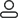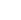LanguageLogin|Sign up
Blog >> Blog Details Page
Thermal Design of PCB Surface Mount Power Devices
Posted:03:22 PM May 09, 2018 writer: G

1. System requirements:

VOUT = 5.0V; VIN (MAX) = 9.0V; VIN (MIN) = 5.6V; IOUT = 700mA; Operating cycle = 100%; TA = 50 °C According to the above system requirements select 750mA MIC2937A-5.0BU regulator, Its parameters are:

VOUT = 5V ± 2% (worst case when overheated) TJ MAX = 125°C. Using TO-263 package, θJC=3°C/W; θCS≈0°C/W (directly soldered on circuit board).

2. Preliminary calculation:

VOUT(MIN)=5V-5×2%=4.9VPD=(VIN(MAX)-VOUT(MIN))+IOUT+(VIN(MAX)×I)=[9V-4.9V]×700mA+(9V×15mA) = 3W maximum value of temperature rise, ΔT = TJ(MAX) - TA = 125°C - 50°C = 75°C; thermal resistance θJA (worst case): ΔT/PD = 75°C/3.0W = 25°C/W.

Thermal resistance of the heat sink, θSA=θJA-(θJC+θCS); θSA=25-(3+0)=22°C/W (maximum).

3. Determine the physical dimensions of the radiator:

A square, single-sided, horizontal solder mask with a copper foil heatsink and a heat-dissipating copper foil covered with a black oil paint, with a 1.3 m/sec air cooling solution, is better than the latter.

With the solid-line approach, a conservative design requires 5,000mm2 of heat-dissipating copper foil, a 71mm x 71mm (2.8 inches on each side) square.

4. Thermal requirements for SO-8 and SOT-223 packages:

Calculate the thermal dissipation area under the following conditions: VOUT=5.0V; VIN(MAX)=14V; VIN(MIN)=5.6V; IOUT=150mA; Duty Cycle=100%; TA=50°C. Circuit board production equipment is more likely to handle dual-in-line SO-8 packaged devices under permissible conditions. Can SO-8 meet this requirement? With the MIC2951-03BM (SO-8 package), the following parameters can be obtained:

TJ MAX=125°C; θJC≈100°C/W.

5. Calculate the parameters using the SO-8 package:

PD = [14V-5V] x 150mA + (14V x 8mA) = 1.46W; elevated temperature = 125°C - 50°C = 75°C; thermal resistance θJA (worst case):

ΔT/PD = 75°C/1.46W = 51.3°C/W; θSA = 51-100 = -49°C/W (maximum).

Obviously, SO-8 can not meet the design requirements without refrigeration. Consider the MIC5201-5.0BS regulator in the SOT-223 package. The package is smaller than the SO-8, but its three pins have good heat dissipation. Select MIC5201-3.3BS, its related parameters are as follows:

TJ MAX=125°C The thermal resistance of OT-223 is θJC=15°C/WθCS=0°C/W (directly soldered on the board).

6. Calculate the result of using SOT-223 package:

PD = [14V-4.9V] × 150mA + (14V × 1.5mA) = 1.4W rising temperature = 125°C - 50°C = 75°C; thermal resistance θJA (worst case):

ΔT/PD=75°C/1.4W=54°C/W; θSA=54-15=39°C/W (maximum). According to the above data, referring to Figure 1, the use of 1,400 mm2 heat-dissipating copper foil (a square with a side length of 1.5 inches) can meet the design requirements.

The above design results can be used as a rough reference. The actual design needs to understand the thermal characteristics of the circuit board and obtain more accurate results that meet the actual design.

949 1 0 1
• PCB
Prototype
• PCB
Assembly
• SMD
Stencil
 Dimensions: (mm) × Quantity: (pcs) 5 5 10 15 20 25 30 40 50 75 100 120 150 200 250 300 350 400 450 500 600 700 800 900 1000 1500 2000 2500 3000 3500 4000 4500 5000 5500 6000 6500 7000 7500 8000 9000 10000 Other Quantities:(quantity*length*width is greater than 10㎡) OK Layers: 1 2 4 6 8 10 12 Thickness: 0.6 mm 0.8 mm 1.0 mm 1.2 mm 1.6 mm 2.0 mm 2.5 mmQuote now